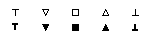EDIS | Guide | FAQ | New | Search | Bibliography | Index | Feedback | About |# XDI State Graph

The following definitions are adapted from [Verhoeff94]. For conventions used in figures of XDI state graphs see below.

## Definitions

An XDI state graph is a directed graph, decorated in several ways, defining the external structure and observable behavior of a system.

An arrow takes on one of the following two appearances:[ zoom | xfig ]
Moreover, every arrow is labeled with a terminal identifier. Dashed arrows are called input arrows, representing the occurrence of an input signal on the associated terminal. Similarly, solid arrows are called output arrows, representing the occurrence of an output signal on the associated terminal.

A node takes on one of the following ten appearances:[ zoom | xfig ]
From left to right, the five shapes are referred to as: top, transient (or nabla), indifferent (or box), demanding (or delta), and bottom. The bold filled versions on the lower line are used for the initial state. Here is an interpretation of the node shapes:

• Top nodes represent a miracle state (to be avoided by the system).
• Transient nodes represent states with an output obligation (to be fulfilled by the system when no input is received).
• Indifferent nodes represent states with neither input nor output obligation,
• Demanding nodes represent states with an input obligation (to be fulfilled by the environment) but no output obligation.
• Bottom nodes represent an error state (to be avoided by the environment).

Finally, there are some syntactic restrictions on XDI state graphs:

1. Arrows are labeled consistently (w.r.t. appearance); that is, every terminal identifier either appears on input arrows only, or on output arrows only.
2. There is exactly one initial node (bold filled node).
3. For every node, the arrows leaving that node have distinct labels.
4. All nodes have identical sets of outgoing arrow labels.
5. Every node is reachable from the initial node via a path of zero or more arrows.
6. A transient node has at least one outgoing output arrow.
7. A demanding node has at least one outgoing input arrow.
8. An input arrow to a top node comes from a top node.
9. An output arrow to a bottom node comes from a bottom node.
10. Arrows from a top node only go to a top node.
11. Arrows from a bottom node only go to a bottom node.
12. They are minimal as explained below.
13. They satisfy the Extended JTU Rules.
The input alphabet of an XDI state graph is the set of labels appearing on input arrows. Similarly, the output alphabet is the set of labels appearing on output arrows. On account of restriction 1, the input and output alphabets are disjoint. The alphabet of the state graph is the union of its input and output alphabet.

Restrictions 2 and 3 expresses that the state graph is unambiguous. Restriction 4 expresses that the state graph is complete. Restriction 5 expresses that the state graph is connected.

### Minimality

Consider a node p and a string s over the state graph's alphabet. On account of restrictions 3 and 4, there is a unique node p/s reachable from p via a path of arrows spelling out s. For the purpose of this explanation, let us define F.p.s=`shape of p/s'. An XDI state graph is said to be minimal when for all distinct nodes p and q there exists a string s such that F.p.s differs from F.q.s.

## Figures of XDI state graphs

### Implied arrows and nodes

Because of restrictions 10 and 11, top and bottom nodes are "sink" states. That is why top and bottom nodes, and arrows pointing to them, are usually omitted in figures of XDI state graphs. However, we will always leave enough arrows so that the alphabets can be still be deduced correctly from the figure.

As a consequence of leaving certain arrows and nodes implicit, a figure of an XDI state graph appears to violate restriction 4. "Missing" arrows and nodes can be reconstructed as follows. Whenever a node lacks a certain outgoing input arrow this arrow should be added going to a bottom node, which should be added if not already present. Similarly, whenever a node lacks a certain outgoing output arrow this arrow should be added going to a top node, which should be added if not already present.

### Implied arrow labels

To avoid clutter in figures of XDI state graphs, some arrow labels may have been omitted. Two parallel arrows of the same appearance usually (should) have the same labels. Furthermore, the Extended JTU Rules help in determining missing arrow labels.

### Node numbers

In figures of XDI state graphs, nodes are numbered for reference purposes. The initial state is usually given number 0. These numbers are not part of the state graph.

Nodes with the same number are one and the same node (having the same appearance in all occurrences) and all their incoming and outgoing arrows should be "taken together". An arrow pointing to just a number is understood to point to the node with that number (such a node should appear somewhere in the figure).

## XDI format

The overall structure of a file in XDI format is:
```Name:
<name>
Terminals:
<terminal list>
InitialState:
<state>
States+Arrows:
( <state> <state label> <transition list> )*
```
where
```  <terminal list> ::= <empty>
| <terminal> <direction> <terminal list>
<terminal> ::= <identifier>
<direction> ::= ? | !
<state> ::= <identifier>
<state label> ::= > | # | <
<transition list> ::= <empty>
| <terminal> : <state> <transition list>
```
Notes:
?
labels an input terminal
!
labels an output terminal
>
labels a transient state
#
labels an indifferent state
<
labels a demanding state

Each state is labeled by a unique identifier, usually a number. The label of the bottom state is `BOT` and of the top state it is `TOP`.